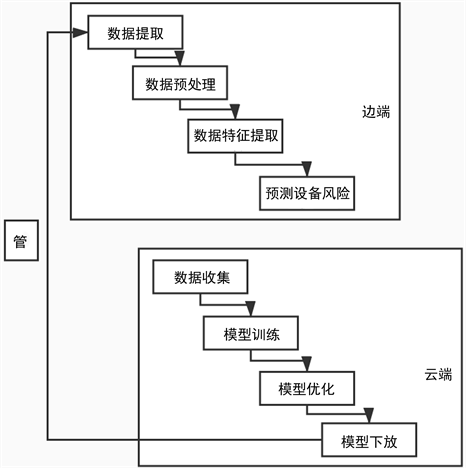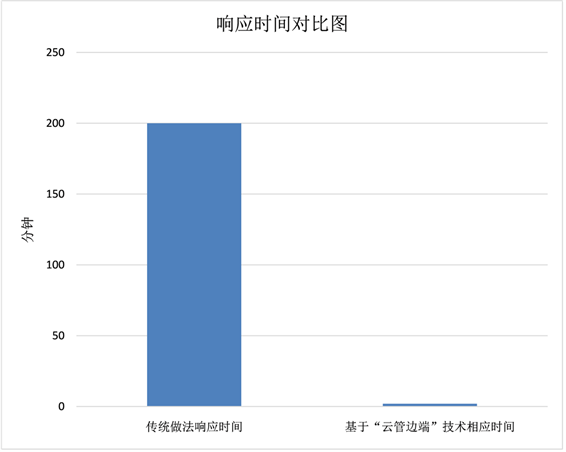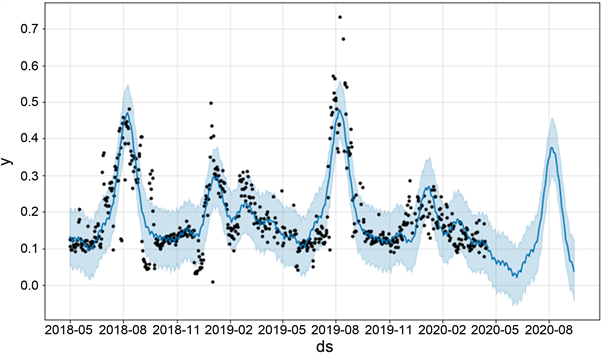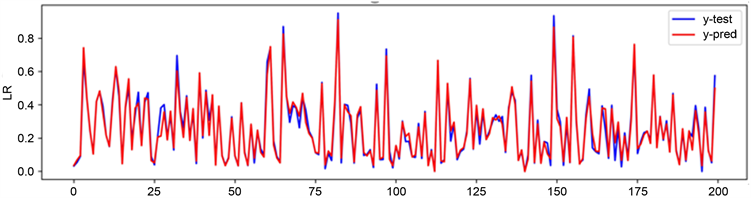#### 期刊菜单

A Research on Equipment Risk Prevention Based on “Cloud Tube Edge End” Technology

Abstract: In the context of the gradual increase in the number of users connected to power distribution station and the increasing importance of power equipment risk prevention, the necessity of risk prevention for power distribution station has gradually emerged. Although there has been certain research and practice on equipment risk prevention, however, in the process of practice, there is still no efficient solution for the large number of equipment, wide range, and complex data in the power field. This paper proposes a risk prevention method for power distribution station based on the “cloud-network-edge-end” technology. Based on the method described in this article, the efficiency and accuracy of risk prevention can be significantly improved.

1. 引言

2. 背景

3. 技术及方案分析

3.1. 云管边端

“云管边端”和云计算的区别主要是云计算中心/平台只储存数据，处理数据和传输数据，云计算中心/平台是数据的使用者；但是边缘计算因为靠近用户侧，处于网络的边缘位置，分布式的数据处理中心/平台不仅存储、处理、传输数据，更重要的是，在边缘计算架构下，数据处理中心/平台还是数据的生产者。这种将核心网用户面功能下沉到边缘侧的架构减少了网络的重复操作，降低了数据请求传输到响应请求的时延，提高了用户的服务质量和体验。合理的架构还可以增加提供的数据信息量、缓解网络拥塞的程度，并为用户带去更多的应用和更佳的服务 。

3.2. 设备风险防范

$O{b}_{i}^{\left(t\right)}={\sum }_{i=1}^{n}l\left({y}_{i},{\stackrel{^}{y}}_{i}^{\left(t-1\right)}+{f}_{t}\left({x}_{i}\right)\right)+\Omega \left({f}_{t}\right)+\text{constant}$ (1)

$Ob{j}^{\left(t\right)}\approx {\sum }_{i=1}^{n}\left[l\left({y}_{i},{\stackrel{^}{y}}_{i}^{\left(t-1\right)}\right)+{g}_{i}{f}_{t}\left({x}_{i}\right)+\frac{1}{2}{h}_{i}{f}_{t}^{2}\left({x}_{i}\right)\right]+\Omega \left({f}_{t}\right)+\text{constant}$ (2)

${g}_{i}={\partial }_{{\stackrel{^}{y}}^{\left(t-1\right)}}l\left({y}_{i},{\stackrel{^}{y}}^{\left(t-1\right)}\right)$ (3)

${h}_{i}={\partial }_{{\stackrel{^}{y}}^{\left(t-1\right)}}^{2}l\left({y}_{i},{\stackrel{^}{y}}^{\left(t-1\right)}\right)$ (4)

1) 使用近似算法来判断最佳分割点；

2) 不仅支持以CART作为基分类器，Xgboost还支持线性分类器；

3) 对于特征的值有缺失的样本，Xgboost可以自动学习出它的分裂方向；

4) 可在系统设计模块，块结构设计等进行并行化处理；

5) 针对cache和内存做了优化；

Xgboost的预测准确性取决于特征选取的是否全面，本文在特征构建的过程中，构建了：1) 设备属性特征，包括设备额定容量、总装接容量、设备投运时间以及基于设备属性等基本属性特征，以及衍生出来如设备负荷趋势类型等特征；2) 历史数据特征，包括整体分布特征、趋势增长特征、周期性特征、波动程度特征等基于历史数据构建的特征；3) 外部环境特征：包括日期类型特征、气象特征等与设备风险相关联的外部特征。相比于原始的设备层面的特征，本文基于“云管边端”技术的应用，在特征选择方面充分考虑了特征的全面性。

3.3. 方案设计

4. 结果分析

1) 从对设备风险防范的效率上来看，采用传统云端的方法，对浦东供电公司2万个台区的实际用时为200分钟，而采用本文所述的“云管边端”技术的响应时间为分钟级(图2)。可以发现，传统云端的不能实时对设备风险进行防范，而基于“云管边端”技术则可以实现对设备风险的实时监控及防范。Figure 1. Flow chart of Equipment Risk Prevention Based on “Cloud Tube Edge

End” TechnologyFigure 2. Example of Response time comparison

2) 对设备风险判断的准确性，传统做法通过对两万个台区的数据进行融合，预处理，统一建模分析，经过多次的模型训练以及优化模型，风险预测的结果如图3所示：其中均方根误差(RMSE)为：0.1，平均绝对误差(MAE)为0.07，R2为0.67，如果以R2作为模型预测结果好坏的参考标准，采用传统做法，对于两万个台区的风险预测准确性可以达到70%。Figure 3. Example diagram of the results of risk predictionFigure 4. Result of power distribution station load prediction

5. 结语

  陆冰芳, 张希翔. 基于机器学习的电网信息系统安全风险预测模型构建[J]. 电子设计工程, 2020, 28(13): 128-132.  熊小敏, 杨鑫, 刘兆璘, 朱雪田. 车路协同的云管边端架构及服务研究[J]. 电子技术应用, 2019, 45(8): 14-18+31.  徐亚兰, 郭承军. 基于边缘计算的高精度地图数据处理方法研究现状[C]//第十一届中国卫星导航年会. 论文集S02导航与位置服务, 2020: 96-101.